# AND Function

Determines if the given conditions in a test are TRUE

## What is the AND Function?

The AND function is categorized under Excel Logical functions. It is used to determine if the given conditions in a test are TRUE. For example, we can use the function to test if a number in cell B1 is greater than 50 and less than 100.

As a financial analyst, the function is useful in testing multiple conditions, specifically, if multiple conditions are met to make sure they all are true. Also, it helps us avoid extra nested IFS, and the AND function can be combined with the OR function.

### Formula

=AND(logical1, [logical2], …)

The AND function uses the following arguments:

1. Logical1 (required argument) – This is the first condition or logical value to be evaluated.
2. Logical2 (optional requirement) – This is the second condition or logical value to be evaluated.

#### Notes:

1. We can set up to 255 conditions for the AND function.
2. The function tests a number of supplied conditions and returns:
1. TRUE if ALL of the conditions evaluate to TRUE; or
2. FALSE otherwise (i.e., if ANY of the conditions evaluate to FALSE).

### How to Use the AND Function in Excel?

To understand the uses of the AND function, let us consider a few examples:

#### Example 1

Suppose we wish to calculate the bonus for every salesperson in our company. To be eligible for a 5% bonus, the salesperson should have achieved sales higher than \$5,000 in a year. Otherwise, they should have achieved an account goal of 4 accounts or higher. To earn a bonus of 15%, they should have achieved both conditions together.

We are given the data given below: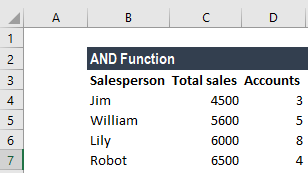To calculate the bonus commission, we will use the AND function. The formula will be: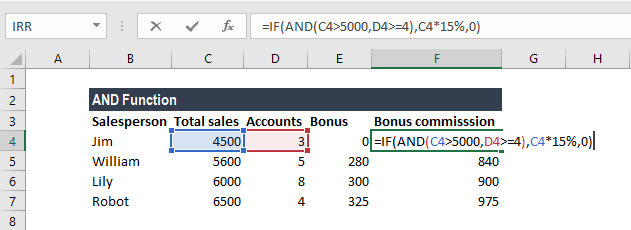The formula for calculating the bonus does not require the AND function but we need to use the OR function. The formula to use will be: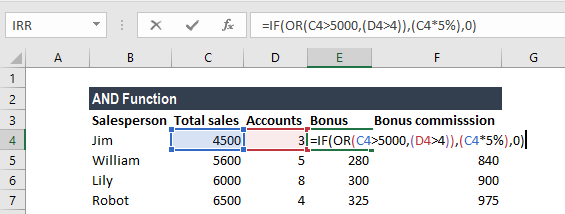The resulting table will be similar to the one below: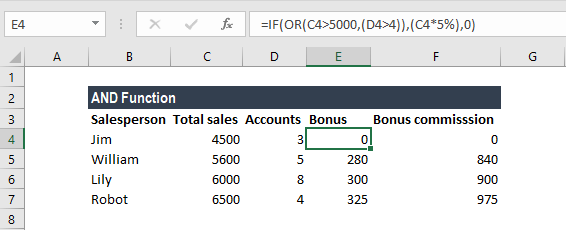#### Example 2

Let’s see how we can use the AND function to test if a numeric value falls between two specific numbers. For this, we can use the AND function with two logical tests.

Suppose we are given the data below: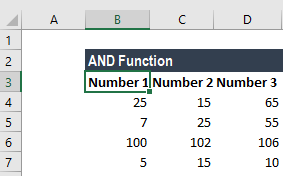The formula to use is: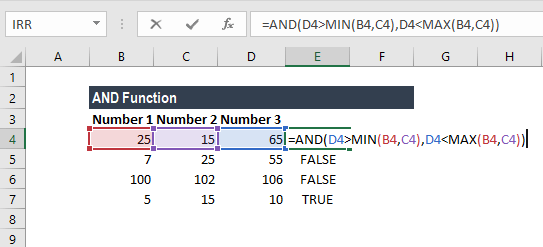In the above formula, the value is compared to the smaller of the two numbers, determined by the MIN function. In the second expression, the value is compared to the larger of the two numbers, determined by the MAX function. The AND function will return TRUE only when the value is greater than the smaller number and less than the larger number.

We get the results below: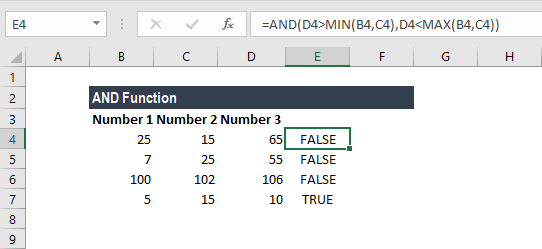### Things to Remember about the AND Function

1. VALUE! error – Occurs when no logical values are found or created during the evaluation.
2. Test values or empty cells provided as arguments are ignored by the AND function.

Thanks for reading CFI’s guide to important Excel functions! By taking the time to learn and master these functions, you’ll significantly speed up your financial analysis. To learn more, check out these additional CFI resources: